﻿ Practice Math Questions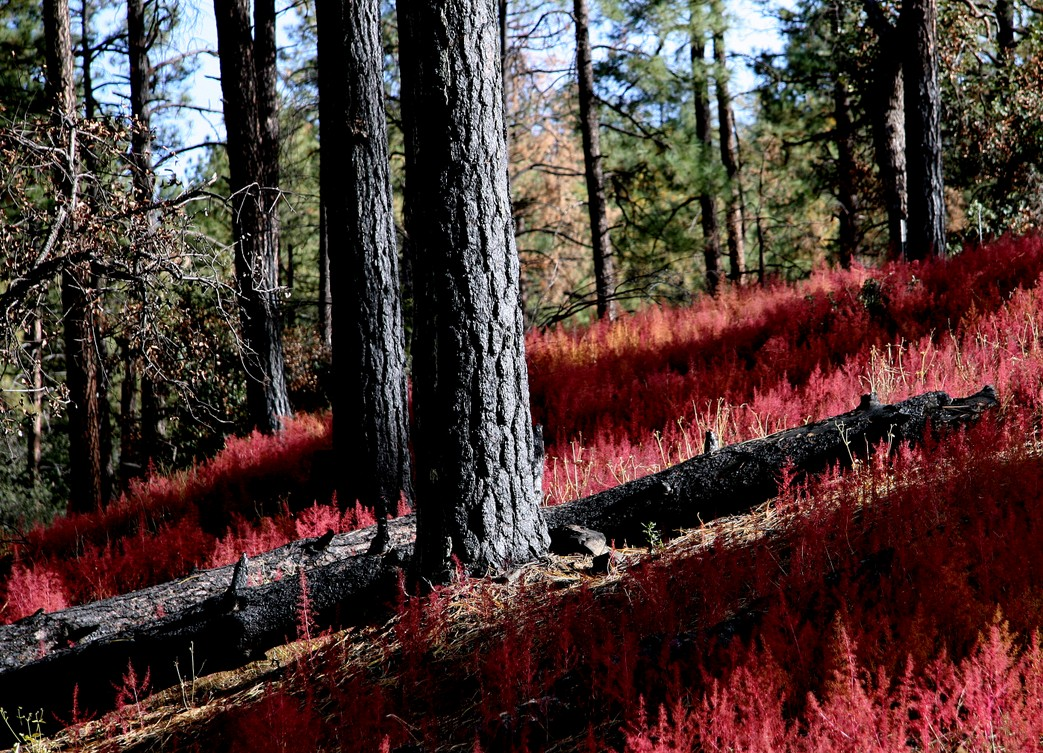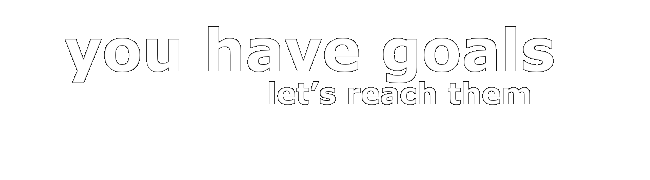# Practice Math Questions

• Try these on for size.

 1) What's the radius of the largest sphere that can be placed inside a cube of volume 64?   (A) 16 (B) 8 (C) 4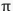(D) 2(E) 2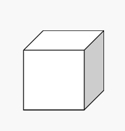2) One side of this square is on the radius of the circle. The circle's radius is 6. What's the area of the blue region?   (A) 12(B) 36(C) 36- 12 (D) 36 - 9(E) 36- 9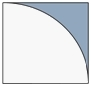• 3) The average of 12 consecutive even integers is 981. What's the highest of these integers?
•
• (A) 988
• (B) 989
• (C) 990
• (D) 991
• (E) 992
•

•
• 1) What's the radius of the largest sphere that can be placed inside a cube of volume 64?
•
•
• 1. If the cube's volume is 64, then each edge has a length of 4.
•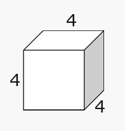•
• 2. The largest circle or sphere that fits inside this cube has a diameter of 4.
•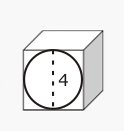•
• 3. The radius is half the diameter, so the radius is 2.
•

 2) One side of this square is on the center of the circle. The circle's radius is 6. What's the area of the blue region?   Answer is (D) 36 - 9• 1. The area of the square is 36.
••
• 2. The area of the entire circle is 36.
•
•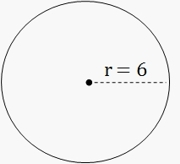•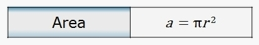•
• 3. Only 1/4 of the circle is in the drawing. 1/4 of 36is 9.
•
•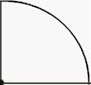•
•
• 4. Subtract the area of the quarter circle from the area of square.
•
••
• 5. The area of the blue region is 36 - 9•
• The answer is typically in terms of.
•

• 3) The average of 12 consecutive even integers is 981. What's the highest of these integers?
•
•
• 1. There are 12 numbers, so draw 12 spaces.
•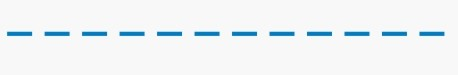•
• 2. The average is 981, which goes right in the middle.
•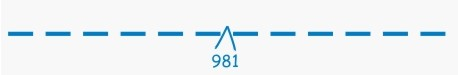•
• 3. The consecutive numbers are even, so we know what the next number is.
•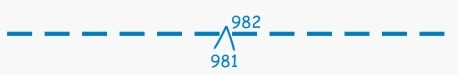•
• 4. And from there, we know what all the other numbers are.
•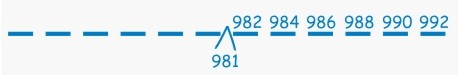•
• 5. The highest is 992.